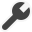ReportField.TotalType

The total type for the field:

• "N" = no totaling
• "S" = sum
• "C" = count
• "B" = count distinct
• "A" = average
• "L" = lowest
• "H" = highest
• "D" = standard deviation
• "V" = variance
`public string TotalType { get; set; }`